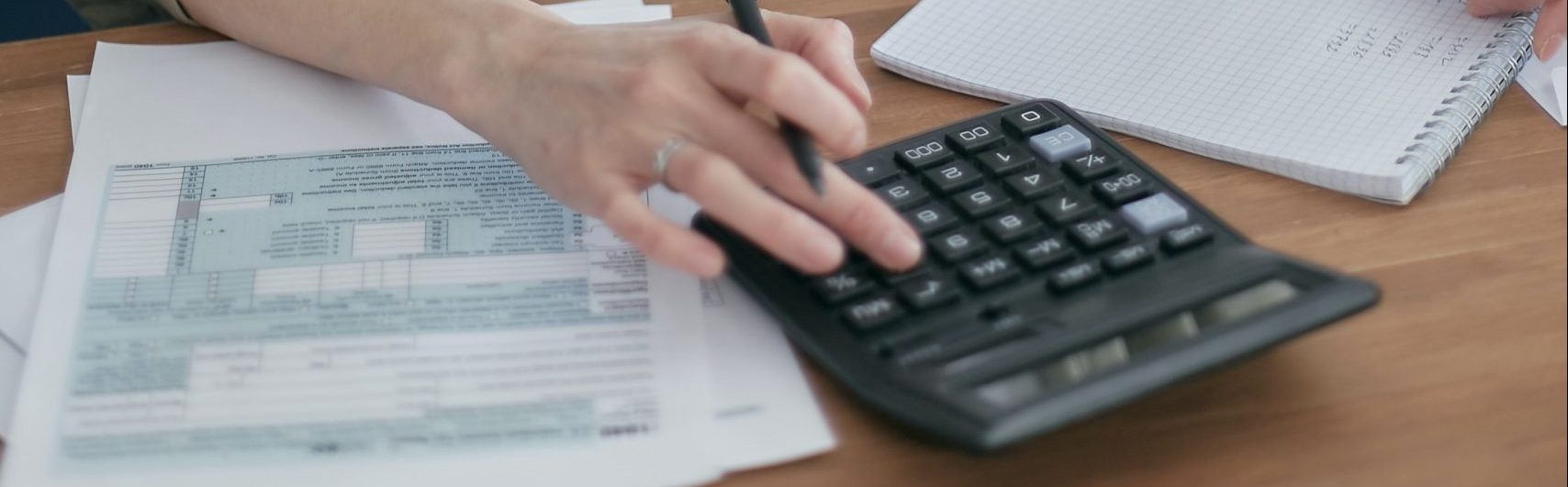### How do calculators compute square roots?

In school, we all learn how to multiply add and divide numbers. They are simple algorithms and it is easy to conceive how computers perform the same kind of calculations. And this is mostly what happens: instead of decimals, a … Read More### Units

How fast does a mouse move, if it ran 50 yards in 2 minutes? In other calculator apps, you have to enter the following: (50*0.9144/1000)/(2/60) -> 1.37 First, you have to convert yards to meters, then to kilometers, then divide … Read More